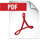# MALDI Application: Structural analysis of anionic surfactants in MALDI negative ion mode using "SpiralTOF™-plus"

• Summary

## MSTips No.333

The matrix-assisted laser desorption ionization time-of-flight mass spectrometer (MALDI-TOFMS) is a powerful tool in analyzing polymers.
Since MALDI mainly produces singly charged ions, m/z values in the mass spectrum are the same as polymer ions' mass. Using the high mass resolution MALDI-TOFMS, it is possible to identify the compositions of polymer series by their repeating unit and the end-group, and calculate the molecular weight distributions of each. The Kendrick Mass Defect (KMD) was recently applied to the polymer analysis to visualize polymer series contained in complex high-mass resolution mass spectra. In addition, the TOF-TOF option enables structural analysis, especially for end-group analysis, of polymers from fragment ions generated by high-energy collision-induced dissociation (HE-CID). In polymer analysis with MALDI-TOFMS, sample, matrix and cationizing agent solution are mixed, dropped onto a target plate, air-dried, and measured. In general, the target polymer ions will be observed in the positive ion mode as [M+Li]+, [M+Na]+ or [M+K]+ according to the cationizing agents. However, polymers that have sulfate or phosphate end-groups can be detected in the negative ions. In this report, we will show the analysis of the mixture of anionic and nonionic surfactants.

## Experiment

The sample used was a detergent containing alkyl ether sulfate (AES) and polyoxyethylene alkyl ether (POEAE) diluted 100-fold with methanol. A saturated methanol solution of α-cyano-4-hydroxysilicate (CHCA) was used for the matrix, and a 1 mg / mL tetrahydrofuran solution of sodium trifluoroacetate (NaTFA) was used as the cationizing agent. In the case of measurement in the positive ion mode, NaTFA solution was spotted first, and then a 1: 1 (v / v) mixture of the sample solution and the matrix solution was spotted and air-dried. In the negative ion mode measurement, a solution in which the sample solution and the matrix solution were mixed at a ratio of 1: 5 (v / v) was spotted and air-dried. The JMS-S3000's SpiralTOF positive/negative ion modes were used to acquire the mass spectrum, and the TOF-TOF negative ion mode was used to acquire the product ion spectrum. The msRepeatFinder was used for KMD analysis.

## Measurement result: Positive ion mode

Figure 1 shows (a) mass spectrum and (b) KMD plot (Base unit C2H4O) in positive ion mode. It can be seen that many components are observed in the mass spectrum of figure 1a. The separation of isobaric peaks with Δm 0.13u could be confirmed even in m/z 575 using high mass resolution MALDI-TOFMS. As a result of composition estimation from accurate mass analysis, the red and green arrows were estimated as POEAE and AES, respectively. The composition of AES was estimated as RO-(EO)n-SO3Na+Na+（R=C12H25, C14H29, EO is ethylene oxide).
When the mass spectrum of the figure. 1a was visualized in KMD plot (figure 1b), two groups (colored with green and red) could be identified.
The red and green groups are POEAE and AES, respectively. A polymers series containing elements with large mass defects are appeared in the upper side of the KMD plot due to the large KMD values. Therefore, AES containing sulfate (SO3Na) end-groups were plotted on the upper side of KMD plot. Using the KMD plot is advantageous, which can easily separate the polymer groups containing end-groups with significantly different elemental compositions in the complex mass spectrum.## Measurement result: Negative ion mode

Figure 2 shows (a) mass spectrum and (b) KMD plot (Base unit C2H4O) in negative ion mode. In negative ion mode, only two polymer series were observed. As a result of the composition estimation, it was estimated that RO-(EO)n-SO3-（R=C12H25, C14H29, EO is ethylene oxide). It was found that AES can be selectively observed when measured in the negative ion mode. In addition, the product ion spectrum of m/z 777.5, 793.5 selected from each series were acquired by TOF-TOF mode. In the product ion spectrum, ions were observed at m/z 80, 97, which correspond to SO3- and SO4H-, suggesting that both series have a sulfate end-group. In addition, 198u and 170u neutral losses were observed from product ion spectra of m/z 777.5 and 793.5, respectively. They corresponded to C14H30 and C12H26, suggesting that the other end-groups are alkyl chains.

## Summary

The AES was observed as RO-(EO)n-SO3Na+Na+ in the positive ion mode. If the sample containing AES and POEAE, the mass spectrum will be complicated because both of them can be ionized in the positive ion mode. On the other hand, AES was selectively ionized in the negative ion mode. Furthermore, end-groups were estimated by TOF-TOF negative ion mode. It was found that if an anionic surfactant such as AES is contained in the sample, it is worth trying to confirm their existence and perform structural analysis using negative ion mode.## Figure 2　Negative ion mass spectrum (a) and KMD plot (b) of detergent containing AES and POEAE.## Figure 3　Negative ion product ion mass spectra of two types of AESs.